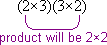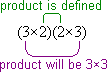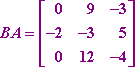Search

Matrix Multiplication Defined (page 2 of 3)Just as with adding matrices, the sizes of the matrices matter when we are multiplying. For matrix multiplication to work, the columns of the second matrix have to have the same number of entries as do the rows of the first matrix.

 AB =If, using the above matrices, B had had only two rows, its columns would have been too short to multiply against the rows of A. Then "AB" would not have existed; the product would have been "undefined". Likewise, if B had had, say, four rows, or alternatively if A had had two or four columns, then AB would not have existed, because A and B would not have been the right sizes.

In other words, for AB to exist (that is, for the very process of matrix multiplication to be able to function sensibly), A must have the same number of columns as B has rows; looking at the matrices, the rows of A must be the same length as the columns of B.

You can use this fact to check quickly whether a given multiplication is defined. Write the product in terms of the matrix dimensions. In the case of the above problem, A is 2×3 and B is 3×2, so AB is (2×3)(3×2). The middle values match:...so the multiplication is defined. By the way, you will recall that AB, the product matrix, was 2×2. You can also see this on the dimensions:Using this, you can see that BA must be a different matrix from AB, because:The product BA is defined (that is, we can do the multiplication), but the product, when the matrices are multiplied in this order, will be 3×3, not 2×2. In particular, matrix multiplication is not "commutative"; you cannot switch the order of the factors and expect to end up with the same result. (You should expect to see a "concept" question relating to this fact on your next test.)

• Given the following matrices, find the product BA.
•The multiplication works the same way as the previous problem, going across the rows and down the columns. I won't try drawing my hands again, but you can see the computations in the colors below:   Copyright © Elizabeth Stapel 2003-2011 All Rights ReservedMatrix multiplication is probably the first time that the Commutative Property has ever been an issue. Remember when they made a big deal, back in middle school or earlier, about how "ab = ba" or "5×6 = 6×5"? That "rule" probably seemed fairly stupid at the time, because you already knew that order didn't matter in multiplication. Introducing you to those rules back then was probably kind of pointless, since order didn't matter for anything you were multiplying then. Well, now the Law of Commutativity does matter, because order does matter for matrix multiplication. Always keep in mind that, for matrices, AB almost certainly does not equal BA.

<< Previous  Top  |  1 | 2 | 3  |  Return to Index  Next >>

 Cite this article as: Stapel, Elizabeth. "Matrix Multiplication Defined." Purplemath. Available from     https://www.purplemath.com/modules/mtrxmult2.htm. Accessed [Date] [Month] 2016

Study Skills Survey

Tutoring from Purplemath
Find a local math tutor